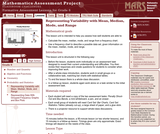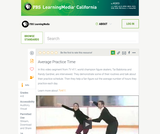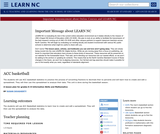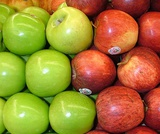Updating search results...

# 18 Results

View
Selected filters:
• CCSS.Math.Content.6.SP.B.5aOnly Sharing Permitted
CC BY-NC-ND
Rating
0.0 stars

This lesson unit is intended to help you assess how well students are able to: Calculate the mean, median, mode, and range from a frequency chart; and to use a frequency chart to describe a possible data set, given information on the mean, median, mode, and range.

Subject:
Education
Mathematics
Physics
Material Type:
Activity/Lab
Assessment
Lesson Plan
Provider:
Shell Center for Mathematical Education
Provider Set:
Mathematics Assessment Project (MAP)
Author:
Shell Center Team
Date Added:
01/17/2013Read the Fine Print
Educational Use
Rating
0.0 stars

The CyberSquad searches for Hackerë_í__ castle based on a survey of where town residents have last seen him in this video from Cyberchase.

Subject:
Mathematics
Material Type:
Lecture
Provider:
PBS LearningMedia
Provider Set:
PBS Learning Media: Multimedia Resources for the Classroom and Professional Development
Author:
U.S. Department of Education
WNET
Date Added:
09/22/2008Read the Fine Print
Educational Use
Rating
0.0 stars

In this video segment from TV411, figure skaters compute their average daily practice time.

Subject:
Mathematics
Material Type:
Lecture
Provider:
PBS LearningMedia
Provider Set:
PBS Learning Media: Multimedia Resources for the Classroom and Professional Development
Author:
U.S. Department of Education
WNET
Date Added:
07/10/2008Conditional Remix & Share Permitted
CC BY-NC-SA
Rating
0.0 stars

The students will use ACC basketball statistics to practice the process of converting fractions to decimals then to percents and will learn how to create and edit a spreadsheet. They will then use this spreadsheet to analyze their data. This unit is done during the basketball season which takes approximately 15 weeks from the middle of November to the middle of March. Teachers must have Clarisworks to open the sample spreadsheet in the lesson, but may recreate it in another spreadsheet program.

Subject:
Statistics and Probability
Material Type:
Lesson Plan
Provider:
University of North Carolina at Chapel Hill School of Education
Provider Set:
LEARN NC Lesson Plans
Author:
Susan Dougherty
Date Added:
07/14/2000Read the Fine Print
Educational Use
Rating
4.0 stars

In this activity, students determine their own eyesight and calculate what a good average eyesight value for the class would be. Students learn about technologies to enhance eyesight and how engineers play an important role in the development of these technologies.

Subject:
Engineering
Material Type:
Activity/Lab
Provider:
TeachEngineering
Provider Set:
TeachEngineering
Author:
Denali Lander
Janet Yowell
Joe Freidrichsen
Malinda Schaefer Zarske
Date Added:
09/26/2008Conditional Remix & Share Permitted
CC BY-NC
Rating
0.0 stars

Lesson OverviewStudents complete a card sort that requires them to match sets of statistics with the corresponding line plots.Students match cards with simple line plots to the corresponding card with measures of center. Some cards include mode, mean, median, and range, and some have one or two measures missing.  Students discuss how they determined which cards would match.Key ConceptsTo complete the card sort in this lesson efficiently, students must be able to relate statistical measures with line plots. If they start with the measures that are easy to see, they can narrow down the possible matches.The mode is the easiest measure to see immediately. It is simply the number with the tallest column of dots.The range can be found easily by subtracting the least value in the plot from the greatest.The median can be found fairly quickly by counting to the middle dot or by pairing dots on the ends until reaching the middle.The mean must be calculated by adding data values and dividing.Goals and Learning ObjectivesApply knowledge of measures of center and range to solve problems.Discuss and review strategy choices when problem solving.

Subject:
Statistics and Probability
Material Type:
Lesson Plan
Provider:
Pearson
Date Added:
09/21/2015Conditional Remix & Share Permitted
CC BY-NC
Rating
0.0 stars

Students will apply what they have learned in previous lessons to analyze and draw conclusions about a set of data. They will also justify their thinking based on what they know about the measures (e.g., I know the mean is a good number to use to describe what is typical because the range is narrow and so the MAD is low.).Students analyze one of the data sets about the characteristics of sixth grade students that was collected by the class in Lesson 2. Students construct line plots and calculate measures of center and spread in order to further their understanding of the characteristics of a typical sixth grade student.Key ConceptsNo new mathematical ideas are introduced in this lesson. Instead, students apply the skills they have acquired in previous lessons to analyze a data set for one attribute of a sixth grade student. Students make a line plot of the data and find the mean, median, range, MAD, and outliers. They use these results to determine a typical value for their data.Goals and Learning ObjectivesDescribe an attribute of a typical sixth grade student using line plots and measures of center (mean and median) and spread (range and MAD).Justify thinking about which measures are good descriptors of the data set.

Subject:
Statistics and Probability
Material Type:
Lesson Plan
Provider:
Pearson
Date Added:
09/21/2015Conditional Remix & Share Permitted
CC BY-NC
Rating
0.0 stars

Subject:
Mathematics
Material Type:
Full Course
Provider:
Pearson
Date Added:
10/06/2016Read the Fine Print
Rating
0.0 stars

Explore the concept of evaporative cooling through a hands-on experiment. Use a wet cloth and fan to model an air-conditioner and use temperature and relative humidity sensors to collect data. Then digitally plot the data using graphs in the activity. In an optional extension, make your own modifications to improve the cooler's efficiency.

Subject:
Engineering
Education
Mathematics
Chemistry
Physics
Material Type:
Activity/Lab
Diagram/Illustration
Lecture Notes
Provider:
Concord Consortium
Provider Set:
Concord Consortium Collection
Author:
The Concord Consortium
Date Added:
12/12/2011Conditional Remix & Share Permitted
CC BY-NC
Rating
0.0 stars

Students calculate the mean absolute deviation (MAD) for three data sets and use it to decide which data set is best represented by the mean.The concept of mean absolute deviation (MAD) is introduced. Students understand that the sum of the deviation of the data from the mean is zero. Students calculate the MAD and understand its significance. Students find the mean and MAD of a sample set of data.Key ConceptsThe mean absolute deviation (MAD) is a measure of how much the values in a data set deviate from the mean. It is calculated by finding the distance of each value from the mean and then finding the mean of these distances.Goals and Learning ObjectivesGain a deeper understanding of mean.Understand that the mean absolute deviation (MAD) is a measure of how well the mean represents the data.Compare data sets using measures of center (mode, median, mean) and spread (range and MAD).Show that the sum of deviations from the mean is zero.

Subject:
Statistics and Probability
Material Type:
Lesson Plan
Provider:
Pearson
Date Added:
09/21/2015Read the Fine Print
Rating
0.0 stars

Explore a NetLogo model of populations of rabbits, grass, and weeds. First, adjust the model to start with a different rabbit population size. Then adjust model variables, such as how fast the plants or weeds grow, to get more grass than weeds. Change the amount of energy the grass or weeds provide to the rabbits and the food preference. Use line graphs to monitor the effects of changes you make to the model, and determine which settings affect the proportion of grass to weeds when rabbits eat both.

Subject:
Education
Life Science
Ecology
Forestry and Agriculture
Material Type:
Activity/Lab
Data Set
Diagram/Illustration
Lecture Notes
Provider:
Concord Consortium
Provider Set:
Concord Consortium Collection
Author:
The Concord Consortium
Date Added:
01/13/2012Conditional Remix & Share Permitted
CC BY-NC
Rating
0.0 stars

Students make a histogram of their typical-student data and then write a summary of what the histogram shows.Students are introduced to histograms, using the line plot to build them. They investigate how the bin width affects the shape of a histogram. Students understand that a histogram shows the shape of the data, but that measures of center or spread cannot be found from the graph.Key ConceptsA histogram groups data values into intervals and shows the frequency (the number of data values) for each interval as the height of a bar.Histograms are similar to line plots in that they show the shape and distribution of a data set. However, unlike a line plot, which shows frequencies of individual data values, histograms show frequencies of intervals of values.We cannot read individual data values from a histogram, and we can't identify any measures of center or spread.Histograms sometimes have an interval with the most data values, referred to as the mode interval.Histograms are most useful for large data sets, where plotting each individual data point is impractical.The shape of a histogram depends on the chosen width of the interval, called the bin width. Bin widths that are too large or too small can hide important features of the data.Goals and Learning ObjectivesLearn about histograms as another tool to describe data.Show that histograms are used to show the shape of the data for a wider range of data.Compare a line plot and histogram for the same set of data.

Subject:
Statistics and Probability
Material Type:
Lesson Plan
Provider:
Pearson
Date Added:
09/21/2015Conditional Remix & Share Permitted
CC BY-NC
Rating
0.0 stars

In this lesson, students draw a line plot of a set of data and then find the mean of the data. This lesson also informally introduces the concepts of the median, or middle value, and the mode, or most common value. These terms will be formally defined in Lesson 6.Using a sample set of data, students review construction of a line plot. The mean as fair share is introduced as well as the algorithm for mean. Using the sample set of data, students determine the mean and informally describe the set of data, looking at measures of center and the shape of the data. Students also determine the middle 50% of the data.Key ConceptsThe mean is a measure of center and is one of the ways to determine what is typical for a set of data.The mean is often called the average. It is found by adding all values together and then dividing by the number of values.A line plot is a visual representation of the data. It can be used to find the mean by adjusting the data points to one value, such that the sum of the data does not change.Goals and Learning ObjectivesReview construction of a line plot.Introduce the concept of the mean as a measure of center.Use the fair-share method and standard algorithm to find the mean.

Subject:
Statistics and Probability
Material Type:
Lesson Plan
Provider:
Pearson
Date Added:
09/21/2015Conditional Remix & Share Permitted
CC BY-NC
Rating
0.0 stars

Students explore how adjusting the bin width or adding, deleting, or moving data values affects a histogram.Students use the Histogram interactive to explore how the bin width can affect how the data are displayed and interpreted. Students also explore how adjusting the line plot affects the histogram.Key ConceptsAs students learned in the last lesson, a histogram shows data in intervals. It shows how much data is in each bin, but it does not show individual data. In this lesson, students will see that the same histogram can be made with different sets of data. Students will also see that the bin width can greatly affect how the histogram looks.Goals and Learning ObjectivesExplore what the shape of the histogram tells us about the data set and how the bin width affects the shape of the histogram.Clarify similarities and differences between histograms and line plots.Compare a line plot and histogram for the same set of data.

Subject:
Statistics and Probability
Material Type:
Lesson Plan
Provider:
Pearson
Date Added:
09/21/2015Conditional Remix & Share Permitted
CC BY-NC
Rating
0.0 stars

In this lesson, students are given criteria about measures of center, and they must create line plots for data that meet the criteria. Students also explore the effect on the median and the mean when values are added to a data set.Students use a tool that shows a line plot where measures of center are shown. Students manipulate the graph and observe how the measures are affected. Students explore how well each measure describes the data and discover that the mean is affected more by extreme values than the mode or median. The mathematical definitions for measures of center and spread are formalized.Key ConceptsStudents use the Line Plot with Stats interactive to develop a greater understanding of the measures of center. Here are a few of the things students may discover:The mean and the median do not have to be data points.The mean is affected by extreme values, while the median is not.Adding values above the mean increases the mean. Adding values below the mean decreases the mean.You can add values above and below the mean without changing the mean, as long as those points are “balanced.”Adding values above the median may or may not increase the median. Adding values below the median may or may not decrease the median.Adding equal numbers of points above and below the median does not change the median.The measures of center can be related in any number of ways. For example, the mean can be greater than the median, the median can be greater than the mean, and the mode can be greater than or less than either of these measures.Note: In other courses, students will learn that a set of data may have more than one mode. That will not be the case in this lesson.Goals and Learning ObjectivesExplore how changing the data in a line plot affects the measures of center (mean, median).Understand that the mean is affected by outliers more than the median is.Create line plots that fit criteria for given measures of center.

Subject:
Statistics and Probability
Material Type:
Lesson Plan
Provider:
Pearson
Date Added:
09/21/2015Rating
0.0 stars

Distributions and Variability

Type of Unit: Project

Prior Knowledge

Students should be able to:

Represent and interpret data using a line plot.
Understand other visual representations of data.

Lesson Flow

Students begin the unit by discussing what constitutes a statistical question. In order to answer statistical questions, data must be gathered in a consistent and accurate manner and then analyzed using appropriate tools.

Students learn different tools for analyzing data, including:

Measures of center: mean (average), median, mode
Measures of spread: mean absolute deviation, lower and upper extremes, lower and upper quartile, interquartile range
Visual representations: line plot, box plot, histogram

These tools are compared and contrasted to better understand the benefits and limitations of each. Analyzing different data sets using these tools will develop an understanding for which ones are the most appropriate to interpret the given data.

To demonstrate their understanding of the concepts, students will work on a project for the duration of the unit. The project will involve identifying an appropriate statistical question, collecting data, analyzing data, and presenting the results. It will serve as the final assessment.

Subject:
Mathematics
Statistics and Probability
Material Type:
Unit of Study
Provider:
PearsonRead the Fine Print
Rating
0.0 stars

Many factors influence the success and survival rate of a population of living things. Explore several factors that can determine the survival of a population of sheep in this NetLogo model. Start with a model of unlimited grass available to the sheep and watch what happens to the sheep population! Next try to keep the population under control by removing sheep periodically. Change the birthrate, grass regrowth rate, and the amount of energy rabbits get from the grass to keep a stable population.

Subject:
Life Science
Ecology
Forestry and Agriculture
Material Type:
Activity/Lab
Data Set
Diagram/Illustration
Lecture Notes
Provider:
Concord Consortium
Provider Set:
Concord Consortium Collection
Author:
The Concord Consortium
Date Added:
01/13/2012Conditional Remix & Share Permitted
CC BY-NC
Rating
0.0 stars

Students make a box plot for their typical-sixth-grader data from Lesson 7 and write a summary of what the plot shows.Using the line plot from Lesson 4, students construct a box plot. Students learn how to calculate the five-number summary and interquartile range (IQR). Students apply this knowledge to the data used in Lesson 7 and describe the data in terms of the box plot. Class discussion focuses on comparing the two graphs and what they show for the sets of data.Key ConceptsA box-and-whisker plot, or box plot, shows the spread of a set of data. It shows five key measures, called the five-number summary.Lower extreme: The smallest value in the data setLower quartile: The middle of the lower half of the data, and the value that 25% of the data fall belowMedian: The middle of the data setUpper quartile: The middle of the upper half of the data, and the value that 25% of the data are aboveUpper extreme: The greatest value in the data setThis diagram shows how these values relate to the parts of a box plot.The length of the box represents the interquartile range (IQR), which is the difference between the lower and upper quartile.A box plot divides the data into four equal parts. One quarter of the data is represented by the left whisker, two quarters by each half of the box, and one quarter by the right whisker. If one of these parts is long, the data in that quarter are spread out. If one of these quarters is short, the data in that quarter are clustered together.Goals and Learning ObjectivesLearn how to construct box plots, another tool to describe data.Learn about the five-number summary, interquartile range, and how they are related to box plots.Compare a line plot and box plot for the same set of data.

Subject:
Statistics and Probability
Material Type:
Lesson Plan
Provider:
Pearson
Date Added:
09/21/2015